GeeksforGeeks App
Open AppBrowser
Continue

## Related Articles

• NCERT Solutions for Class 9 Maths

# Class 9 NCERT Solutions- Chapter 4 Linear Equations in two variables – Exercise 4.3

### Question 1. Draw the graph of each of the following linear equations in two variables:

(i) x + y = 4

(ii) x – y = 2

(iii) y = 3x

(iv) 3 = 2x + y

Solution:

(i) To draw the graph x+y=4, we need at least two solutions of the equation.

We can check that when, x = 0, y = 4,

and x = 4, y = 0

are solutions of the given equation. So, we can use the following table to draw the graph: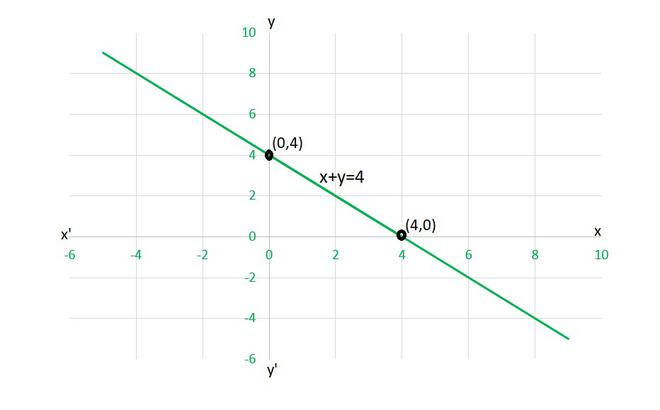(ii) To draw the graph x-y=2, we need at least two solutions of the equation.

We can check that when, x = 0, y = -2,

and x = 2, y = 0

are solutions of the given equation. So, we can use the following table to draw the graph: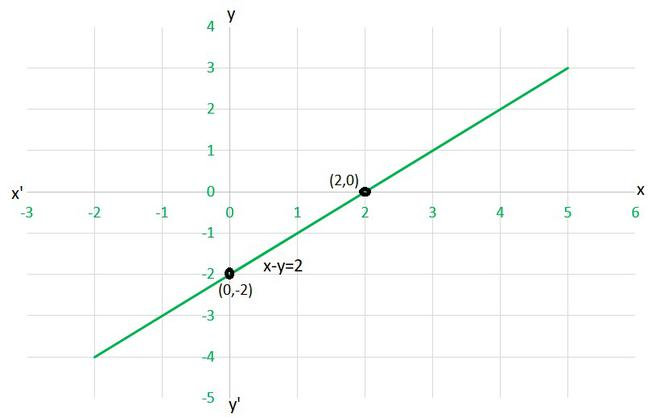(iii) To draw the graph y=3x, we need at least two solutions of the equation.

We can check that when, x = 0, y =0,

and x = 1, y = 3

are solutions of the given equation. So, we can use the following table to draw the graph :(iv) To draw the graph 3=2x+y, we need at least two solutions of the equation.

We can check that when, x = 0, y =3,

and x = 1, y = 1

are solutions of the given equation. So, we can use the following table to draw the graph :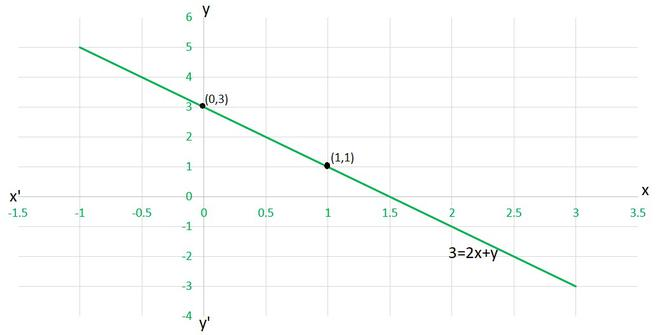### Question 2. Give the equations of two lines passing through (2, 14). How many more such lines are there, and why?

Solution:

Here, as given point is (2,14) so

x=2 and y=14

We can form various equation such as,

y-x = 14-2 = 12

x+y = 2+14 =16

2x+y = 4+14 = 18

y-2x = 14-4 = 10

y = 14

x = 2

and many more……..

In fact, there are infinitely many linear equations which are satisfied by the coordinates of the point (2, 14).

As it is stated that there can be infinite lines passing through a point. So here,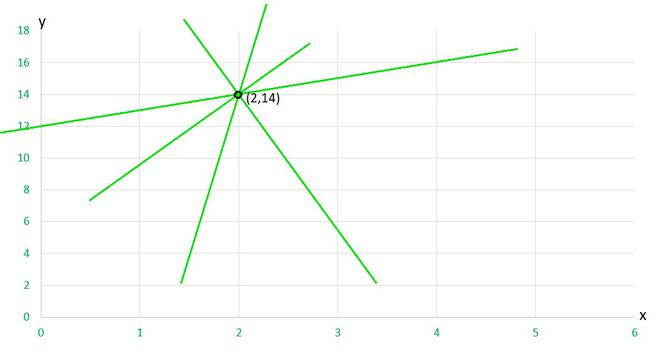### Question 3. If the point (3, 4) lies on the graph of the equation 3y = ax + 7, find the value of a.

Solution:

The given equation is

3y = ax+7

According to the given point (3,4),

x = 3 and y = 4

As this point lie on this graph, then it will satisfy these points. So substituting the values

3y = ax+7

⟹ (3×4) = (a×3)+7

12 = 3a+7

3a = 12–7

3a = 5

a =Hence, the value of a =.

### Question 4. The taxi fare in a city is as follows: For the first kilometer, the fare is ₹ 8 and for the subsequent distance it is ₹ 5 per km. Taking the distance covered as x km and total fare as ₹ y, write a linear equation for this information, and draw its graph.

Solution:

So, as given in the question, we will take

Distance covered as x km

and total fare as ₹ y

Fare for the first kilometer = 8 per km

Fare after the first 1km = ₹ 5 per km

Let x is the total distance, then the distance after one km will be = (x-1)km

Hence., Fare after the first km = 5(x-1)

So, in equation form we can conclude that,

The total fare = Fare of first km+ fare after the first km

y = 8+5(x-1)

y = 8+5(x-1)

y = 8+5x – 5

y = 5x+3

To draw the graph y=5x+3, we need at least two solutions of the equation.

We can check that when, x = 0, y =3,

and x = -1, y = -2

are solutions of the given equation. So, we can use the following table to draw the graph :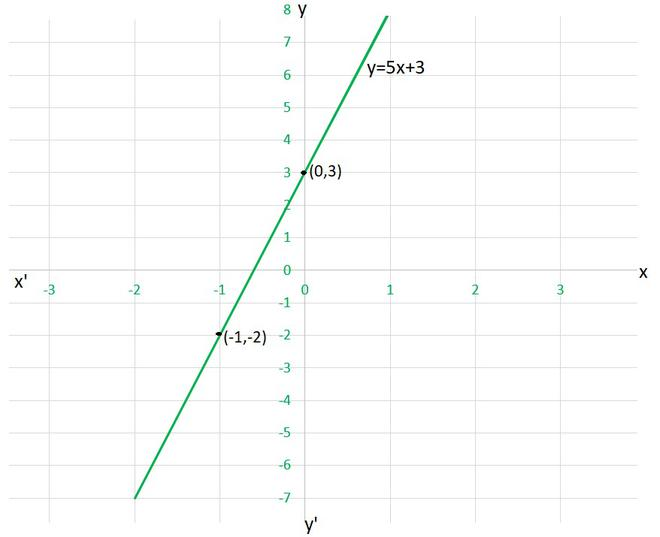### Question 5. From the choices given below, choose the equation whose graphs are given in Fig. 4.6 and Fig. 4.7.

For Fig. 4. 6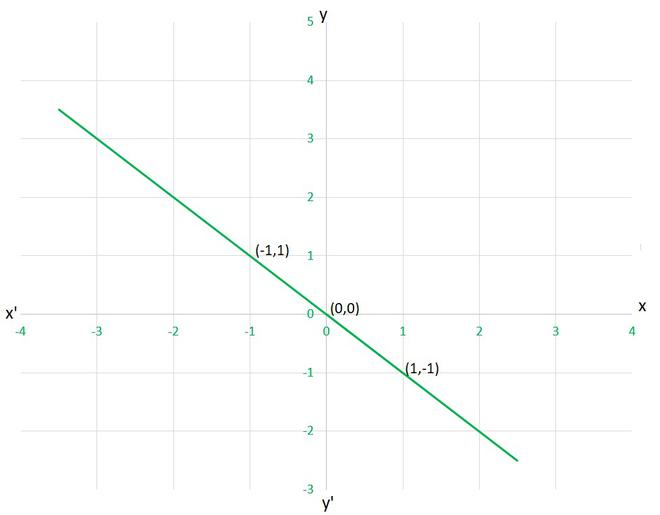(i) y = x

(ii) x + y = 0

(iii) y = 2x

(iv) 2 + 3y = 7x

Solution:

Let’s check each given cases, whether they are satisfying the solution given on graph or not,

(i) y=x

as given point  (1,-1)

x=1 and y=-1 ⟹ x≠y

Hence, this equation is INCORRECT for this graph.

(ii) x+y=0

as given point (1,-1), (1,-1) and (0,0)

x=1 and y=-1 ⟹ x+y=0

x=-1 and y=1 ⟹ x+y=0

x=0 and y=0 ⟹ x+y=0

Hence, this equation is CORRECT for this graph.

(iii) y=2x

as given point  (1,-1)

x=1 and y=-1 ⟹ y≠2x

Hence, this equation is INCORRECT for this graph.

(iii) 2+3y=7x

as given point  (1,-1)

x=1 and y=-1 ⟹ 2+3y≠7x

Hence, this equation is INCORRECT for this graph.

For Fig. 4.7(i) y = x + 2

(ii) y = x – 2

(iii) y = –x + 2

(iv) x + 2y = 6

Solution:

Let’s check each given cases, whether they are satisfying the solution given on graph or not,

(i) y=x+2

as given point (0,2), (2,0) and (-1,3)

x=0 and y=2 ⟹ y=x+2

x=2 and y=0 ⟹ y≠x+2

Hence, this equation is INCORRECT for this graph.

(ii) y=x-2

as given point (0,2)

x=0 and y=2 ⟹ y≠x-2

Hence, this equation is INCORRECT for this graph.

(iii) y=-x+2

as given point (0,2)

x=0 and y=2 ⟹ y=-x+2

x=2 and y=0 ⟹ y=-x+2

x=-1 and y=3 ⟹ y=-x+2

Hence, this equation is CORRECT for this graph.

(iii) x+2y=6

as given point (0,2)

x=0 and y=2 ⟹ x+2y≠6

Hence, this equation is INCORRECT for this graph.

### Question 6. If the work done by a body on application of a constant force is directly proportional to the distance travelled by the body, express this in the form of an equation in two variables and draw the graph of the same by taking the constant force as 5 units. Also read from the graph the work done when the distance travelled by the body is

(i) 2 units

(ii) 0 unit

Solution:

Given in question,

Work done = force × displacement

where constant force = 5 units

lets take work done as y units

and, distance travelled by the body x units

Hence, the equation can be expressed as,

y=5x

To draw the graph y=5x, we need at least two solutions of the equation.

We can check that when, x = 0, y =0,

and x = 1, y = 5

are solutions of the given equation. So, we can use the following table to draw the graph :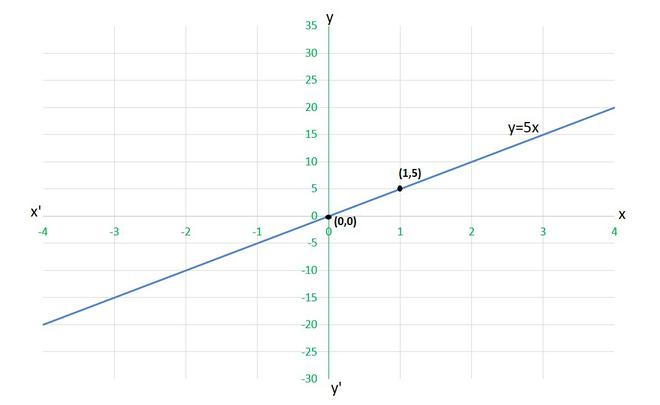(i) for x= 2 units

y=5×2

work done = 10 units

(ii) for x= 0 units

y=5×0

work done = 0 units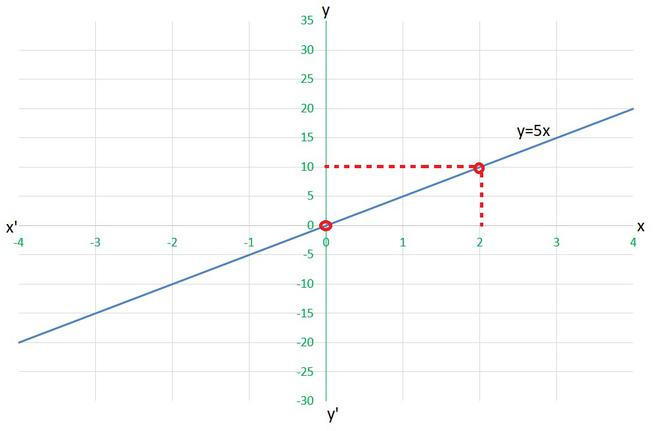### Question 7. Yamini and Fatima, two students of Class IX of a school, together contributed ₹ 100 towards the Prime Minister’s Relief Fund to help the earthquake victims. Write a linear equation that satisfies this data. (You may take their contributions as ₹ x and ₹ y). Draw the graph of the same.

Solution:

Let’s take Yamini’s contribution as ₹ x and Fatima’s contribution as ₹ y.

As they together contributed ₹ 100, so the equation can be formed as,

x+y=100

To draw the graph x+y=100, we need at least two solutions of the equation.

We can check that when, x = 0, y =100,

and x = 100, y = 0

are solutions of the given equation. So, we can use the following table to draw the graph :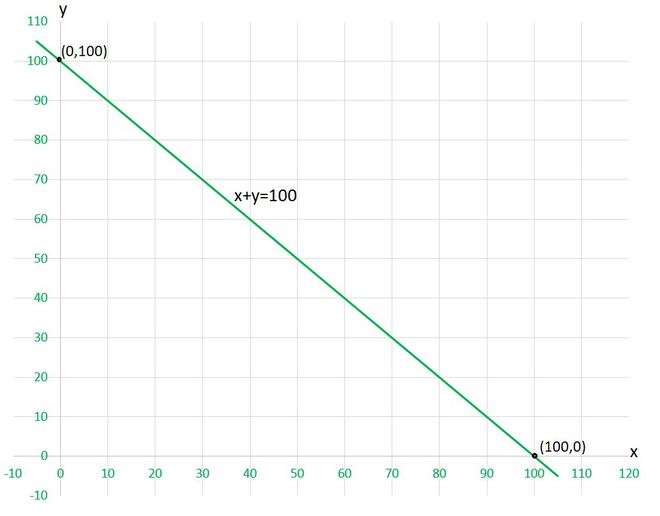### Question 8. In countries like USA and Canada, temperature is measured in Fahrenheit, whereas in countries like India, it is measured in Celsius. Here is a linear equation that converts Fahrenheit to Celsius:

F =C + 32

(i) Draw the graph of the linear equation above using Celsius for x-axis and Fahrenheit for y-axis.

(ii) If the temperature is 30°C, what is the temperature in Fahrenheit?

(iii) If the temperature is 95°F, what is the temperature in Celsius?

(iv) If the temperature is 0°C, what is the temperature in Fahrenheit and if the temperature is 0°F, what is the temperature in Celsius?

(v) Is there a temperature which is numerically the same in both Fahrenheit and Celsius? If yes, find it.

Solution:

(i) Taking Celsius on x-axis and Fahrenheit on y-axis , so equation will be as follows:

y =x + 32

To draw the graph y =x + 32, we need at least two solutions of the equation.

We can check that when, x = 0, y =32,

and x = 5, y = 41

are solutions of the given equation. So, we can use the following table to draw the graph :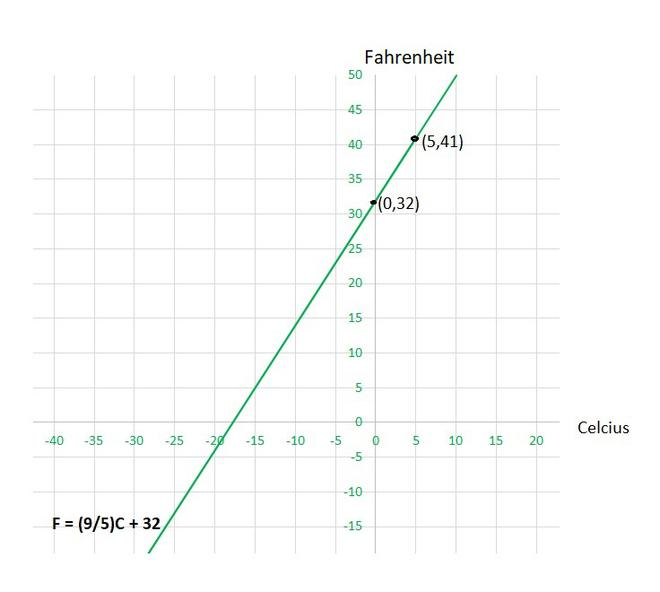(ii) When the temperature is 30°C then, using the equation

y =x + 32

by substituting, x=30, we get

y =×30 + 32

y = 86

Hence, in Fahrenheit temperature will be 86°F

(iii) When the temperature is 95°F then, using the equation

y =x + 32

by substituting, y=95, we get

95 =x+ 32

x =x = 35

Hence, in Celsius temperature will be 35°C

(iv)When the temperature is 0°C, using the equation

y =x + 32

by substituting, x=0, we get

y =×0 + 32

y = 32

Hence, in Fahrenheit temperature will be 32°F

and, when the temperature is 0°F, using the equation and by substituting, y=0, we get

0 =x + 32

x =x = -17.78

Hence, in Celsius temperature will be -17.78°C.

(v) Lets take both temperature same as x.

so, equation becomes as follows:

x =x + 32x-x = -32x = -32

x = -32×x = -40

Hence, Celsius and Fahrenheit temperature will be -40°C and -40°F respectively.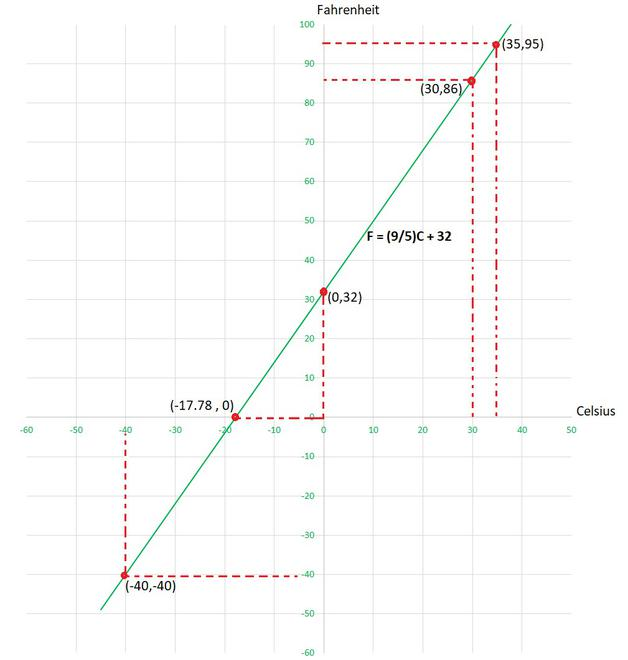My Personal Notes arrow_drop_up
Related Tutorials